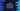# Java string intern method explanation with an example## Java string intern Method :

Java string intern() method is one of the important methods of String class. This method returns an interned string or canonical representation of a string.

If we are using intern() method on a string, it will first check if the same string is available in the memory or not. If available, it will return that string from the memory and if the string is not available, it will create one exact copy of that string object and store it in the String constant pool.

Similarly, if we use the java string intern() method on the same string again, it will return the already available string from the string constant pool.

Using this method, we can ensure that only one copy of a string will be created in the String constant pool.

### Java string intern and equals :

We are invoking the intern() method, equals(Object) method is used internally to check if the String constant pool contains a string equals to our String object. So, if we have two strings str1 and str2, the value of str1.intern() == str2.intern() will be true only if str1.equals(str2) is true.

### Syntax :

The syntax of intern() method is as below :

``````public String intern()
``````
1. This method doesn’t take any parameter.

2. It returns the canonical representation of a string.

### Java string intern method Example :

Let’s try to understand how to string intern works with the below example :

``````public class Example {
public static void main(String[] args) {
String str1 = "Hello";
String str2 = new String("Hello");
String str3 = str2.intern();

//1
System.out.println("str1 == str2 : " + (str1 == str2) + " : " + str1.equals(str2));
//2
System.out.println("str1 == str3 : " + (str1 == str3) + " : " + str1.equals(str3));
//3
System.out.println("str2 == str3 : " + (str2 == str3) + " : " + str2.equals(str3));
}
}
``````

### Output :

``````str1 == str2 : false : true
str1 == str3 : true : true
str2 == str3 : false : true
``````

### Explanation :

If we create one string using the double quotes, it creates that object in the String pool and the object reference points to this area. But if we create using the new keyword, it creates the string in the heap space and also in the String pool but the object points to the heap space. intern() method checks for existence in the String pool.

1. For the first print statement, the result is false. Because str1 is actually pointing to the String pool but str2 is pointing to the Java heap space.

2. The second print statement is true because both are pointing to the same object in the String pool.

3. The last print statement is false because str2 is pointing to the Java heap and str3 is pointing to the String pool.

One thing you should note that the equals method is always returning true here.

### How Java does Automatic string interning :

Whenever we create one String object using a string literal, it creates the string in the string pool and returns the reference. But if the same string is already available in the String pool, it returns that reference instead of creating a new one. Let’s try it with an example :

``````public class Example {
public static void main(String[] args) {
String str1 = "Hello";
String str2 = "Hello";
String str3 = "Hello";
String str4 = "Hello";

System.out.println("str1 == str2 : " + (str1 == str2));
System.out.println("str1 == str3 : " + (str1 == str3));
System.out.println("str1 == str4 : " + (str1 == str4));
System.out.println("str2 == str3 : " + (str2 == str3));
System.out.println("str2 == str4 : " + (str2 == str4));
System.out.println("str3 == str4 : " + (str3 == str4));
}
}
``````

It will print :

``````str1 == str2 : true
str1 == str3 : true
str1 == str4 : true
str2 == str3 : true
str2 == str4 : true
str3 == str4 : true
``````

As you can see that all values are true in this example as all variables are actually pointing to the same string in the memory.

### Conclusion :

We hope that you have found this article helpful. Java String intern is not a commonly used method but it is useful if you are creating a lot of similar variables. Java automatically interns the string literals. For string objects constructed with the new keyword, string literal can be used. You can go through the Oracle string documentation to learn more string methods.

Try to run the examples we have shown above and drop one comment below if you have any queries.